Next: SLA_DMXM - Multiply Matrices
Up: SUBPROGRAM SPECIFICATIONS
Previous: SLA_DMAT - Solve Simultaneous Equations

## SLA_DMOON - Approx Moon Pos/Vel

ACTION:
Approximate geocentric position and velocity of the Moon (double precision).

CALL:
CALL sla_DMOON (DATE, PV)

GIVEN:

 D DATE TDB (loosely ET) as a Modified Julian Date (JD-2400000.5)

RETURNED:

 D(6) PV Moon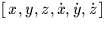, mean equator and equinox of date (AU, AU s-1)

NOTES:
1.
This routine is a full implementation of the algorithm published by Meeus (see reference).
2.
Meeus quotes accuracies of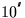in longitude,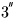in latitude and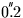arcsec in HP (equivalent to about 20 km in distance). Comparison with JPL DE200 over the interval 1960-2025 gives RMS errors of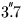and 83 mas/hour in longitude,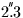arcsec and 48 mas/hour in latitude, 11 km and 81 mm/s in distance. The maximum errors over the same interval are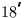and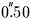/hour in longitude,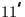and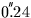/hour in latitude, 40 km and 0.29 m/s in distance.

3.
The original algorithm is expressed in terms of the obsolete timescale Ephemeris Time. Either TDB or TT can be used, but not UT without incurring significant errors (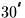at the present time) due to the Moon's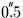/s movement.
4.
The algorithm is based on pre IAU 1976 standards. However, the result has been moved onto the new (FK5) equinox, an adjustment which is in any case much smaller than the intrinsic accuracy of the procedure.
5.
Velocity is obtained by a complete analytical differentiation of the Meeus model.

REFERENCE:
Meeus, l'Astronomie, June 1984, p348.Next: SLA_DMXM - Multiply Matrices
Up: SUBPROGRAM SPECIFICATIONS
Previous: SLA_DMAT - Solve Simultaneous Equations

SLALIB --- Positional Astronomy Library